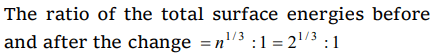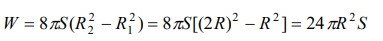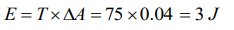## Mechanical Properties of Fluids Questions and Answers Part-9

1. Two small drops of mercury, each of radius R, coalesce to form a single large drop. The ratio of the total surface energies before and after the change is
a) $1 : 2^{1/3}$
b) $2^{1/3} : 1$
c) 2 : 1
d) 1 : 2

Explanation:2.Radius of a soap bubble is increased from R to 2R work done in this process in terms of surface tension is
a) $24\pi R^{2}S$
b) $48\pi R^{2}S$
c) $12\pi R^{2}S$
d) $36\pi R^{2}S$

Explanation:3. The work done in blowing a soap bubble of radius 0.2 m is (the surface tension of soap solution being 0.06 N/m)
a) $192\pi\times10^{-4}J$
b) $280\pi\times10^{-4}J$
c) $200\pi\times10^{-3}J$
d) None of these

Explanation:4. A liquid film is formed in a loop of area 0.05 m2. Increase in its potential energy will be (T = 0.2 N/m)
a) $5\times10^{-2}J$
b) $2\times10^{-2}J$
c) $3\times10^{-2}J$
d) None of these

Explanation:5. In order to float a ring of area $0.04m^{2}$ in a liquidof surface tension 75 N/m, the required surface energy will be
a) 3 J
b) 6.5 J
c) 1.5 J
d) 4 J

Explanation:6. If two soap bubbles of equal radii r coalesce then the radius of curvature of interface between two bubbles will be
a) r
b) o
c) Infinity
d) 1/2r

Explanation:7. A liquid does not wet the sides of a solid, if the angle of contact is
a) Zero
b) Obtuse (More than 90°)
c) Acute (Less than 90°)
d) 90°

Explanation: Obtuse (More than 90°)

8. The meniscus of mercury in the capillary tube is
a) Convex
b) Concave
c) Plane
d) Uncertain

Explanation: The meniscus of mercury in the capillary tube is Convex

9. When the temperature is increased the angle of contact of a liquid
a) Increases
b) Decreases
c) Remains the same
d) First increases and then decreases